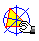CARNOT TRIANGLE

H being the orthocenter of the triangle ABC, we can draw the three triangles ABH, ACH and BCH. The circumcenters C*, B* and A* of these triangles are the vertices of the Carnot triangle of ABC. The Carnot triangle is homothetic and congruent with ABC.Carnot_triangle.mac